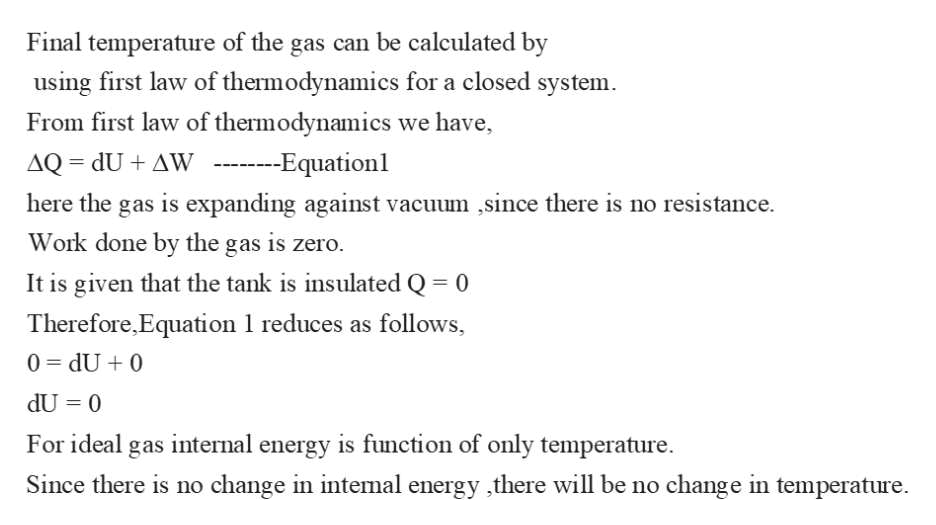# A chamber is divided equally into two parts by a membrane. One side contains Hydrogen, H2, at a pressure of 200 kPa and temperature of 300 K, and the other side is evacuated (a perfect vacuum). The total chamber volume is 0.005 m3. At time t = 0, the membrane ruptures and the hydrogen expands freely into the evacuated side. The chamber can be considered adiabatic (i.e. perfectly insulated). Find the final values of the temperature and pressure.

Question

A chamber is divided equally into two parts by a membrane. One side contains Hydrogen, H2, at a pressure of 200 kPa and temperature of 300 K, and the other side is evacuated (a perfect vacuum). The total chamber volume is 0.005 m3. At time t = 0, the membrane ruptures and the hydrogen expands freely into the evacuated side. The chamber can be considered adiabatic (i.e. perfectly insulated). Find the final values of the temperature and pressure.

check_circleExpert Solution
Step 1

Given information:

Chamber is divided into two equal parts.

Initial pressure  of hydrogen P1 = 200 kPa

Initial temperature T1 = 300 K

Total volume of the chamber is ...help_outlineImage TranscriptioncloseFinal temperature of the gas can be calculated by using first law of thermodynamics for a closed system. From first law of thermodynamics we have AQ dU+ AW --Equationl here the gas is expanding against vacuum ,since there is no resistance. Work done by the gas is zero. It is given that the tank is insulated Q 0 Therefore,Equation 1 reduces as follows, 0 dU 0 dU 0 11 For ideal gas intemal energy is function of only temperature change in temperature Since there is no change in intemal energy ,there will be no fullscreen

### Want to see the full answer?

See Solution

#### Want to see this answer and more?

Solutions are written by subject experts who are available 24/7. Questions are typically answered within 1 hour*

See Solution
*Response times may vary by subject and question
Tagged in

### Thermodynamics